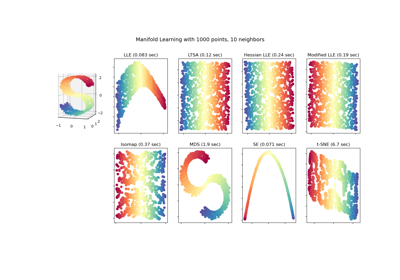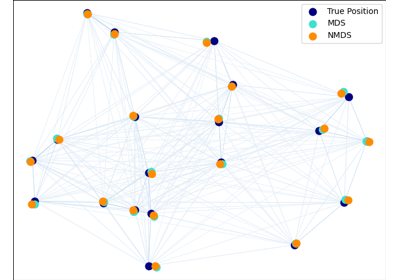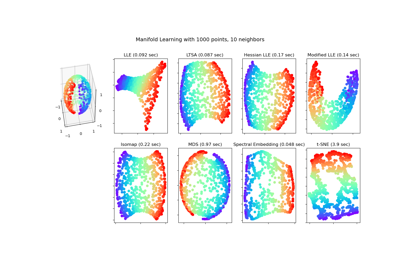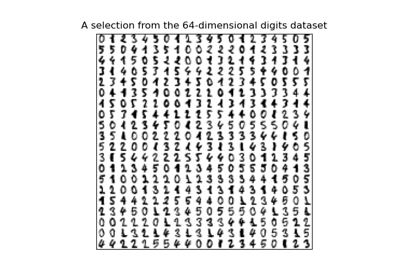# sklearn.manifold.MDS¶

class sklearn.manifold.MDS(n_components=2, *, metric=True, n_init=4, max_iter=300, verbose=0, eps=0.001, n_jobs=None, random_state=None, dissimilarity='euclidean')[source]

Multidimensional scaling

Read more in the User Guide.

Parameters
n_componentsint, optional, default: 2

Number of dimensions in which to immerse the dissimilarities.

metricboolean, optional, default: True

If True, perform metric MDS; otherwise, perform nonmetric MDS.

n_initint, optional, default: 4

Number of times the SMACOF algorithm will be run with different initializations. The final results will be the best output of the runs, determined by the run with the smallest final stress.

max_iterint, optional, default: 300

Maximum number of iterations of the SMACOF algorithm for a single run.

verboseint, optional, default: 0

Level of verbosity.

epsfloat, optional, default: 1e-3

Relative tolerance with respect to stress at which to declare convergence.

n_jobsint or None, optional (default=None)

The number of jobs to use for the computation. If multiple initializations are used (n_init), each run of the algorithm is computed in parallel.

None means 1 unless in a joblib.parallel_backend context. -1 means using all processors. See Glossary for more details.

random_stateint, RandomState instance, default=None

Determines the random number generator used to initialize the centers. Pass an int for reproducible results across multiple function calls. See :term: Glossary <random_state>.

dissimilarity‘euclidean’ | ‘precomputed’, optional, default: ‘euclidean’

Dissimilarity measure to use:

• ‘euclidean’:

Pairwise Euclidean distances between points in the dataset.

• ‘precomputed’:

Pre-computed dissimilarities are passed directly to fit and fit_transform.

Attributes
embedding_array-like, shape (n_samples, n_components)

Stores the position of the dataset in the embedding space.

stress_float

The final value of the stress (sum of squared distance of the disparities and the distances for all constrained points).

References

“Modern Multidimensional Scaling - Theory and Applications” Borg, I.; Groenen P. Springer Series in Statistics (1997)

“Nonmetric multidimensional scaling: a numerical method” Kruskal, J. Psychometrika, 29 (1964)

“Multidimensional scaling by optimizing goodness of fit to a nonmetric hypothesis” Kruskal, J. Psychometrika, 29, (1964)

Examples

>>> from sklearn.datasets import load_digits
>>> from sklearn.manifold import MDS
>>> X.shape
(1797, 64)
>>> embedding = MDS(n_components=2)
>>> X_transformed = embedding.fit_transform(X[:100])
>>> X_transformed.shape
(100, 2)


Methods

 fit(X[, y, init]) Computes the position of the points in the embedding space fit_transform(X[, y, init]) Fit the data from X, and returns the embedded coordinates get_params([deep]) Get parameters for this estimator. set_params(**params) Set the parameters of this estimator.
__init__(n_components=2, *, metric=True, n_init=4, max_iter=300, verbose=0, eps=0.001, n_jobs=None, random_state=None, dissimilarity='euclidean')[source]

Initialize self. See help(type(self)) for accurate signature.

fit(X, y=None, init=None)[source]

Computes the position of the points in the embedding space

Parameters
Xarray, shape (n_samples, n_features) or (n_samples, n_samples)

Input data. If dissimilarity=='precomputed', the input should be the dissimilarity matrix.

yIgnored
initndarray, shape (n_samples,), optional, default: None

Starting configuration of the embedding to initialize the SMACOF algorithm. By default, the algorithm is initialized with a randomly chosen array.

fit_transform(X, y=None, init=None)[source]

Fit the data from X, and returns the embedded coordinates

Parameters
Xarray, shape (n_samples, n_features) or (n_samples, n_samples)

Input data. If dissimilarity=='precomputed', the input should be the dissimilarity matrix.

yIgnored
initndarray, shape (n_samples,), optional, default: None

Starting configuration of the embedding to initialize the SMACOF algorithm. By default, the algorithm is initialized with a randomly chosen array.

get_params(deep=True)[source]

Get parameters for this estimator.

Parameters
deepbool, default=True

If True, will return the parameters for this estimator and contained subobjects that are estimators.

Returns
paramsmapping of string to any

Parameter names mapped to their values.

set_params(**params)[source]

Set the parameters of this estimator.

The method works on simple estimators as well as on nested objects (such as pipelines). The latter have parameters of the form <component>__<parameter> so that it’s possible to update each component of a nested object.

Parameters
**paramsdict

Estimator parameters.

Returns
selfobject

Estimator instance.

## Examples using sklearn.manifold.MDS¶